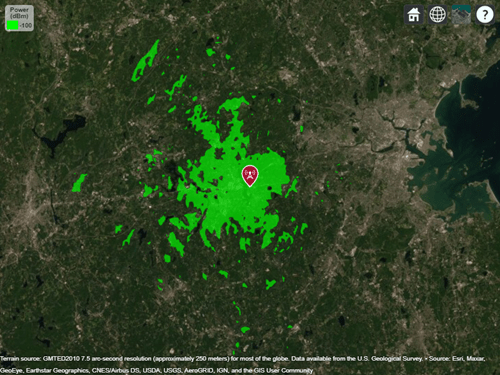TIREM

TIREM propagation model

Description

Model the behavior of electromagnetic radiation from a point of transmission as it travels over irregular terrain, including buildings, by using the Terrain Integrated Rough Earth Model™ (TIREM™) model. Represent the TIREM model by using a TIREM object.

The TIREM model:

• Is valid from 1 MHz to 1000 GHz.

• Calculates path loss from free-space loss, terrain and obstacle diffraction, ground reflection, atmospheric refraction, and tropospheric scatter.

• Provides path loss estimates by combining physics with empirical data.

Creation

Create a TIREM object by using the propagationModel function.

Properties

expand all

Polarization of transmitter and receiver antennas, specified as "horizontal" or "vertical". The object assumes both antennas have the same polarization. The model uses this value to calculate path loss due to ground reflection.

Data Types: char | string

Conductivity of the ground, specified as a numeric scalar in Siemens per meter (S/m) in the range of 0.0005 to 100. The model uses this value to calculate path loss due to ground reflection. The default value corresponds to average ground.

Data Types: double

Relative permittivity of the ground, specified as a numeric scalar in the range of 1 to 100. Relative permittivity is expressed as a ratio of absolute material permittivity to the permittivity of vacuum. The model uses this value to calculate the path loss due to ground reflection. The default value corresponds to average ground.

Data Types: double

Atmospheric refractivity near the ground, specified as a numeric scalar in N-units in the range of 250 to 400. The model uses this value to calculate the path loss due to refraction through the atmosphere and tropospheric scatter. The default value corresponds to average atmospheric conditions.

Data Types: double

Absolute air humidity near the ground, specified as a numeric scalar in grams per cubic meter (g/m3) in the range of 0 to 110. You can use this value to calculate path loss due to atmospheric absorption. The default value corresponds to the absolute humidity of air at 15 degrees Celsius and 70 percent relative humidity.

Data Types: double

Examples

collapse all

Display the coverage area for a transmitter using the TIREM model.

pm = propagationModel("tirem");
tx = txsite("Name","Apple Hill","Latitude",42.3001,"Longitude",-71.3604);
coverage(tx,pm)